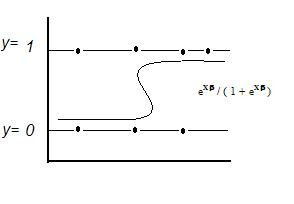## Sunday, September 19, 2010

### Logistic Modeling & Maximum Likelihood Estimation vs. Linear Regression & Ordinary Least Squares

See also: Analysis of the Logistic Function

Ordinary Least Squares:

OLS: y = XB + e

Minimizes the sum of squared residuals e'e where e = (y –XB)

R2 = 1- SSE/SSTOLS With a Dichotomous Dependent Variable: y = (0 or 1)Dichotomous Variables, Expected Value, & Probability:

Linear Regression E[y|X] = XB ‘conditional mean(y) given x‘

If y = { 0 or 1}

E[y|X] = Pi a probability interpretation

Expected Value: sum of products of each possible value a variable can take * the probability of that value occurring.

If P(y=1) = pi and P(y=0) = (1-pi) then E[y] = 1*pi +0* (1-pi) = pithe probability that y=1

Problems with OLS:
1) Estimated probabilities outside (0,1)
2) e~binomial var(e) = n*p*(1-p) violates assumption of uniform variance → unreliable inferences

Logit Model:

Ln ((Di /(1 – Di)) = βX

Di = probability y = 1 = e / ( 1 + e )

Where : Di / (1 – Di) =  ’odds’

E[y|X] = Prob(y = 1|X) = p = e / (1 + e )Maximum Likelihood Estimation

L(θ) =∏f(y,θ) -the product of marginal densities
Take ln of both sides, choose θ to maximize, → θ* (MLE)

Choose θ’s to maximize the likelihood of the sample being observed.Maximizes the likelihood that data comes from a ‘real world’ characterized by one set of θ’s vs another.Estimating a Logit Model Using Maximum Likelihood:

L(β) = ∏f(y, β) = ∏ e / (1 + e ) ∏ 1/(1 + e)

choose β to maximize the ln(L(β)) to get the MLE estimator β*

To get p(y=1) apply the formula Prob( y = 1|X) = p = eXβ* / (1 + eXβ*) utilizing the MLE estimator β*to'score' the data X.

Deriving Odds Ratios:

Exponentiation ( eβ ) gives the odds ratio.

Variance:

When we undertake MLE we typically maximize the log of the likelihood function as follows:

Max Log(L(β)) or LL ‘log likelihood’ or solve:

∂Log(L(β))/∂β = 0     'score matrix'   = u( β)

-∂u(β)/ ∂β    'information matrix'   = I(β)

I-1 (β)   'variance-covariance matrix'   = cramer rao lower bound

Inference:

Wald  χ2  = (βMLE  -β0)Var-1MLE  -β0)

√W ~ t or Z (based on assumptions exact or asymptotic normality)

Assessing Model Fit and Predictive Ability:

Not minimizing sums of squares: R2 = 1 – SSE / SST or SSR/SST. With MLE no sums of squares are produced and no direct measure of R2 is possible. Other measures must be used to assess model performance:

Deviance:   -2 LL where   LL = log-likelihood   (smaller is better)

-2[LL0  - LL1]   L0  = likelihood of  incomplete model  L1 =  likelihood  of more complete model

AIC and SC are deviants of -2LL, and penalize the LL by the # of predictors in the model

Null Deviance:  DN = -2[LLN  - LLp]  LN  = intercept only model  Lp= perfect model ~ SST

Model Deviance: DK = -2[LLK  - LLp]  LK  = intercept only model  Lp= perfect model ~ SSR

Model χ2 : DN -DK For a good fitting model, model deviance will be smaller than null deviance, giving a larger χ2 and a higher level of significance.

Pseudo-r-square:   DN -DK / DN  Smaller (better fitting) DK gives a larger ratio. Not on (0,1)

Cox & Snell Pseudo R square: adjusts for parameters and sample size, not on (0,1)

Nagelkerke (Max-rescaled r-square) :  transformation such that R --> (0,1)

Other:

Percentage of Correct Predictions

Area under the ROC curve:Area = measure of model’s ability to correctly distinguish cases where (y=1) from those that do not based on explanatory variables.

y-axis: sensitivity or prediction that y = 1 when y = 1,
x-axis: 1-specificity or prediction that y = 1 when y = 0, false positive

References:

Menard, Applied Logistic Regression Analysis, 2nd Edition 2002

1.2.